Name:    CP Statistics Chapter 11 Test - Testing a Claim

Multiple Choice
Identify the choice that best completes the statement or answers the question.

1.

An opinion poll asks a random sample of adults whether they favor banning ownership of handguns by private citizens. A radio commentator believes that more than half of all adults favor such a ban. The null and alternative hypotheses you would use to test this claim are
 a) Ho: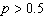;  Ha: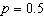b) Ho:;  Ha: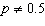c) Ho: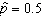;  Ha: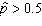d) Ho:;  Ha:e) Ho:;  Ha: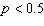2.

DDT is an insecticide that accumulates up the food chain. Predator birds can be contaminated with quite high levels of the chemical by eating many lightly contaminated prey. One effect of DDT upon birds is to inhibit the production of the enzyme carbonic anhydrase, which controls calcium metabolism. It is believed that this causes eggshells to be thinner and weaker than normal and makes the eggs more prone to breakage. (This is one of the reasons why the condor in California is near extinction.) An experiment was conducted where 16 sparrow hawks were fed a mixture of 3 ppm dieldrin and 15 ppm DDT (a combination often found in contaminated prey). The first egg laid by each bird was measured, and the mean shell thickness was found to be 0.19 mm. A “normal” eggshell has a mean thickness of 0.2 mm.

The null and alternative hypotheses are
 a)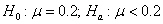b)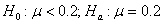c)d)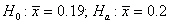e)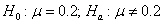3.

A significance test allows you to reject a null hypothesis H0 in favor of an alternative Ha at the 5% significance level. What can you say about significance at the 1% level?
 a) There is sufficient evidence to reject H0 at the 1% significance level. b) Ha can be rejected at the 1% significance level. c) The answer can’t be determined from the information given. d) H0 can be rejected at the 1% significance level. e) There is insufficient evidence to reject H0 at the 1% significance level.

4.

A random sample of 100 likely voters in a small city produced 59 voters in favor of Candidate A. The observed value of the test statistic for testing the null hypothesis H0:versus the alternative Ha:is
 a)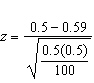b)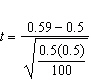c)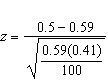d)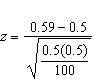e)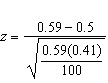5.

We wish to see if the dial indicating the oven temperature for a certain model oven is properly calibrated. Four ovens of this model are selected at random. The dial on each oven is set to 300° F.  After one hour, the actual temperature of each oven is measured. The temperatures observed are 305°, 310°, 300°, and 305° which result in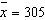and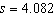. Assuming that the actual temperatures for this model when the dial is set to 300° are normally distributed with mean m, we test whether the dial is properly calibrated by testing the hypotheses   H0: m = 300,   Ha: m ¹ 300.

Based on the data, the value of the one-sample t statistic is
 a) 5 b) 4.90. c) 2.82. d) 2.45. e) 1.23.

6.

Sheldon takes a random sample of 50 U.S. housing units and finds that 30 are owner-occupied. Using a significance test for a proportion, he is not able to reject the null hypothesis that exactly half of U.S. housing units are owner-occupied. Later, Sheldon learns that the U.S. Census for that same year found that 66.2% of housing units are owner-occupied. Select the best description of the type of error in this situation.
 a) A Type I error was made because a false null hypothesis wasn’t rejected. b) A Type I error was made because a false null hypothesis was rejected. c) A Type II error was made because a false null hypothesis wasn’t rejected. d) A Type II error was made because a false null hypothesis was rejected. e) No error was made.

7.

The one sample t statistic from a sample of n = 15 observations for the one-sided test of
H0: m = 8, Ha: m > 8 has the value t = 2.19. Based on this information, which of the following would be true?
 a) 0.05 < P-value < 0.10. b) 0.025 < P-value < 0.05. c) 0.02 < P-value < 0.025. d) 0.01 < P-value < 0.02. e) 0.005 < P-value < 0.01.

8.

Which of the following conditions are not necessary for the use of the one-proportion z procedures?
 a)b)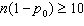c) the data must be a random sample from the population of interest d) The population size is at least 10 times greater than the size of the sample e) All of these conditions are necessary

9.

Which of the following conditions are not necessary for the use of the t procedures?
 a) the population standard deviation is unknown b) the population distribution is Normal or we have a large sample size c) the data must be an SRS from the population d) np0 and n(1 – p0) are both at least 10 e) All of these conditions are necessary

10.

Resting pulse rate is an important measure of the fitness of a person's cardiovascular system, with a lower rate indicative of greater fitness. The mean pulse rate for all adult males is approximately 72 beats per minute. A random sample of 25 male students currently enrolled in the Faculty of Agriculture was selected and the mean resting pulse rate was found to be 80 beats per minute with a standard deviation of 20 beats per minute. The experimenter wishes to test if the students are less fit, on average, than the general population.

A possible Type I error here would be to
 a) conclude that the students are less fit (on average) than the general population when in fact they have equal fitness on average. b) conclude that the students have the same fitness (on average) as the general population when in fact they are less fit (on average). c) conclude that the students have the same fitness (on average) as the general population when in fact they have the same fitness (on average). d) conclude that the students are less fit (on average) than the general population, when, in fact, they are less fit (on average). e) conclude that the students have the same fitness (on average) when in fact they are more fit (on average).